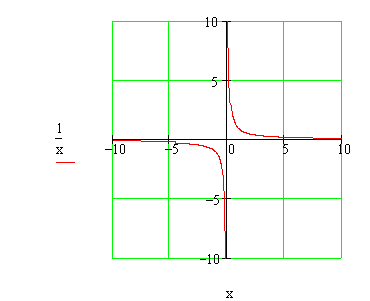Calculus on Demand at Dartmouth College Lecture 6 | Index | Lecture 8 Lecture 7Resources

Math 3 Course Syllabus
Practice Exams

Contents

In this lecture we discuss the concept of the limit of a function at a point, and of the limit of a function at plus infinity or minus infinity.

Quick Question

If we define f(x) = 1/x, is there any value of x for which f(x) = 0?Outline

Outlines for
The Legacy of Galileo, Newton, and Leibniz
Limits of Functions
Limits at Infinity

Textbook

The Legacy of Galileo, Newton, and Leibniz
Limits of Functions
Limits at Infinity

Quiz

The Legacy of Galileo, Newton, and Leibniz Quiz
Limits of Functions Quiz
Limits at Infinity Quiz

Videos

With G(x) a piecewise defined, bumpy function:

Evaluate these limits:

Lecture 6 | Index | Lecture 8